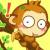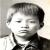# linux c：这个代码有什么问题？行读取和缓冲读取

PYPlus 发布于 2013/07/28 10:54

```static int read_cnt;

static ssize_t
{
{
again:
{
if (errno == EINTR)
goto again;
return (-1);
return (0);
}

return 1;
}

{
ssize_t n,rc;
char c,*ptr;
ptr  = vptr;
for ( n = 1;n < maxlen;n++)
{
{
*ptr ++ = c;
if (c == '\n')
break;
} else if(rc == 0)
{
*ptr =0;
return (n-1);
} else
return (-1);
}
*ptr = 0;
return (n);
}```

```ssize_t readn(int fd,void *vptr,size_t n)
{
size_t nleft;
char *ptr;
ptr = vptr;
nleft = n;
while(nleft > 0)
{
{
if (errno == EINTR)
{
}
else
return -1;
}
break;
}
return (n - nleft);
}```

gcc：gcc (GCC) 4.7.2 20121109 (Red Hat 4.7.2-8)

00#### 引用来自“Zirconi”的答案

000```static int read_cnt = 0;
int er = 0;
er = errno;
if (er != EINTR) {
return 1;
}
}
return 0 ;

}
return 0;
}
}
return 1;
}
return 0;
}

ssizt_t r_readline(int fd ,void *vptr,size_t maxlen){
int i = 0;
if (vptr[i] == '\n'){
i++;
break;
}
i++;
if (i >= maxlen - 1) break;

}
ptr[i] = 0;
return i－1;

}```

char c;